﻿ 基于质点转换和包围盒的混合碰撞检测算法
«上一篇文章快速检索 高级检索

 哈尔滨工程大学学报2018, Vol. 39Issue (10): 1695-1701  DOI: 10.11990/jheu.2017010360

### 引用本文TANG Yuanhao, HOU Jin, WU Tingting, et al. Hybrid collision detection algorithm based on particle conversion and bounding box[J]. Journal of Harbin Engineering University, 2018, 39(10), 1695-1701. DOI: 10.11990/jheu.201701036.### 文章历史

Hybrid collision detection algorithm based on particle conversion and bounding box
TANG Yuanhao, HOU Jin, WU Tingting, GONG Sui, ZHANG Juan, ZHONG Litao
School of Information Science and Technology, Southwest Jiaotong University, Chengdu 611756, China
Abstract: To improve the efficiency of collision detection, this paper presents a collision detection algorithm based on particle conversion and bounding box. The algorithm first makes use of the binary tree and regional center to construct an oriented bounding box (OBB) for all objects in space, then expresses objects separated by a certain distance as a physical particle. The outermost OBB of the object was considered as a dot in a three-dimensional space, so as to calculate the distance between two objects. This distance was determined using the result of dot calculation and distance of reduction, so that the dot that did not pass the test was not detected again, while the dot that passed the validation was reduced. Finally, the OBB intersection test was carried out on the reduced bounding box. The results showed that the proposed algorithm can effectively improve collision detection efficiency compared with its predecessor algorithm, and can further reduce the time and cost of the increased space, especially for the complicated environment where a large number of objects exist in the space.
Keywords: collision detection    regional center    binary tree    OBB hierarchical bounding box    particle conversion    particle reduction

1 质点转换和包围盒混合法总体描述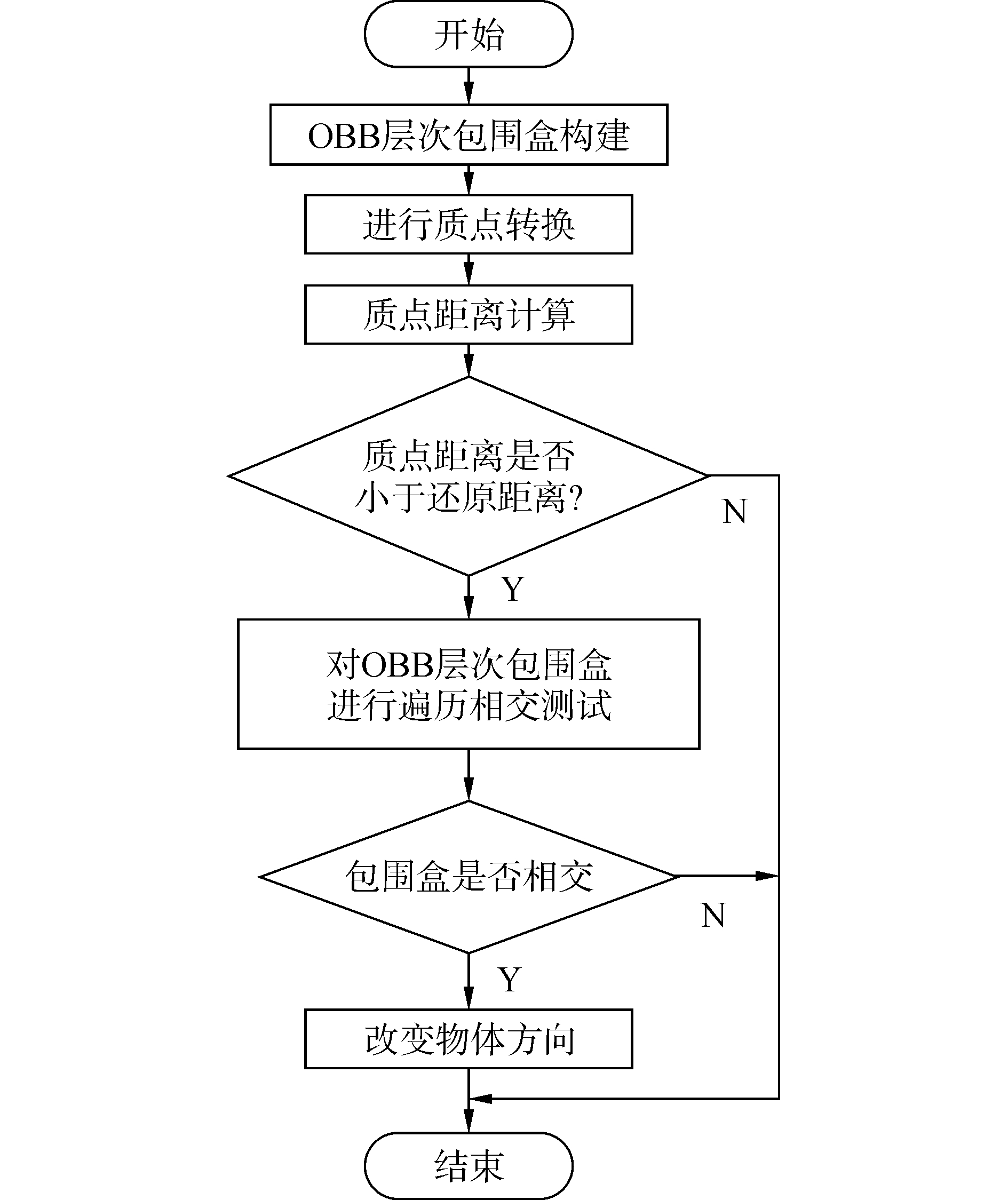Download: 图 1 总体流程图 Fig. 1 Overall flow chart

2 质点转换和包围盒混合法原理 2.1 OBB层次包围盒的构建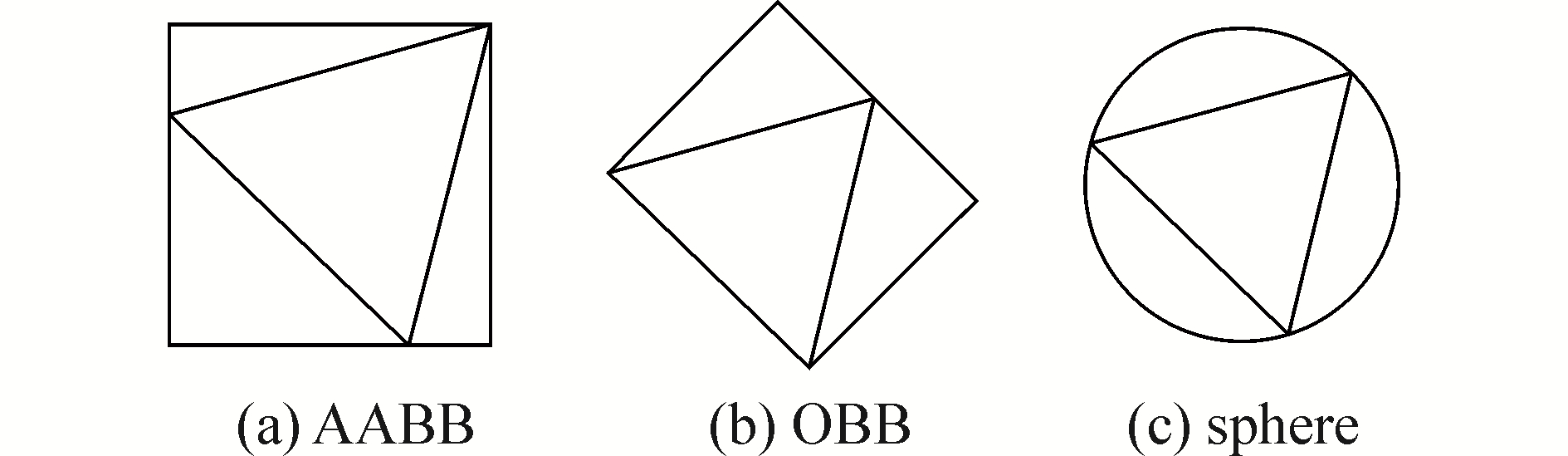Download: 图 2 不同包围盒的构造 Fig. 2 The structure of the different bounding boxes

 $\mathit{\boldsymbol{A}} = \left[ {\begin{array}{*{20}{c}} {{\mathop{\rm cov}} \left( {x, x} \right)}&{{\mathop{\rm cov}} \left( {x, y} \right)}&{{\mathop{\rm cov}} \left( {x, z} \right)}\\ {{\mathop{\rm cov}} \left( {x, y} \right)}&{{\mathop{\rm cov}} \left( {y, y} \right)}&{{\mathop{\rm cov}} \left( {y, z} \right)}\\ {{\mathop{\rm cov}} \left( {x, z} \right)}&{{\mathop{\rm cov}} \left( {y, z} \right)}&{{\mathop{\rm cov}} \left( {z, z} \right)} \end{array}} \right]$ (1)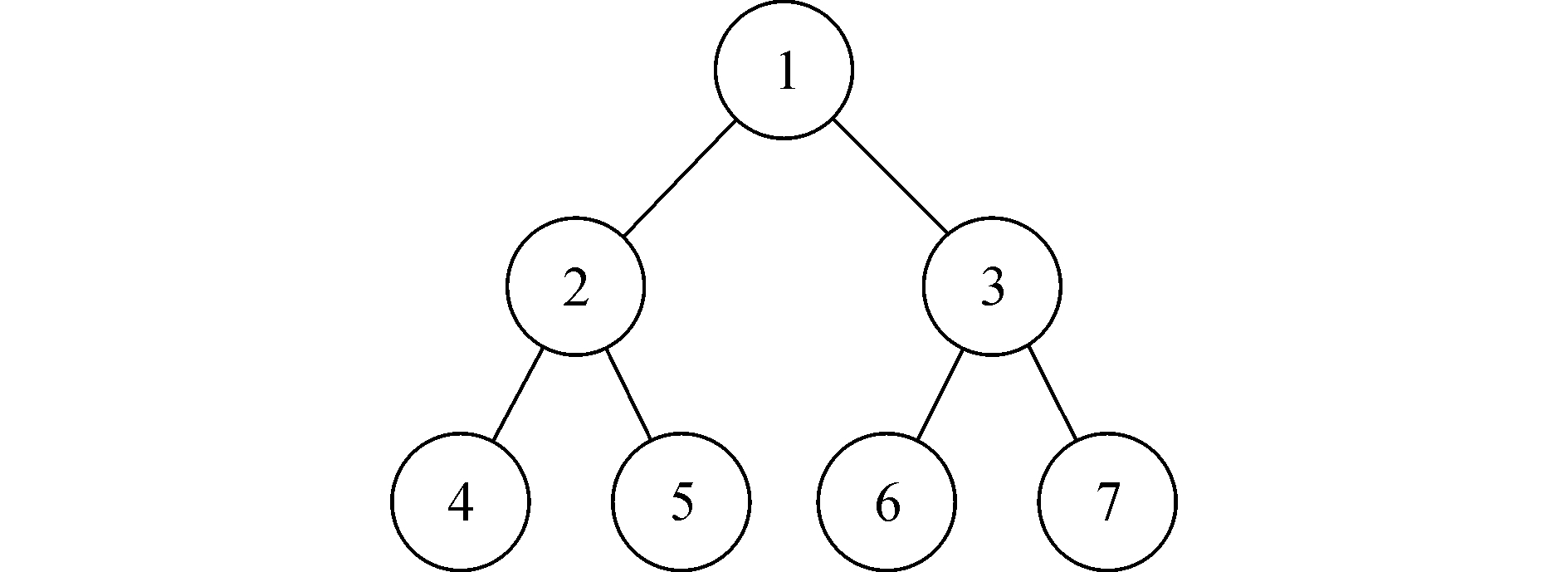Download: 图 3 二叉树原理图 Fig. 3 Binary tree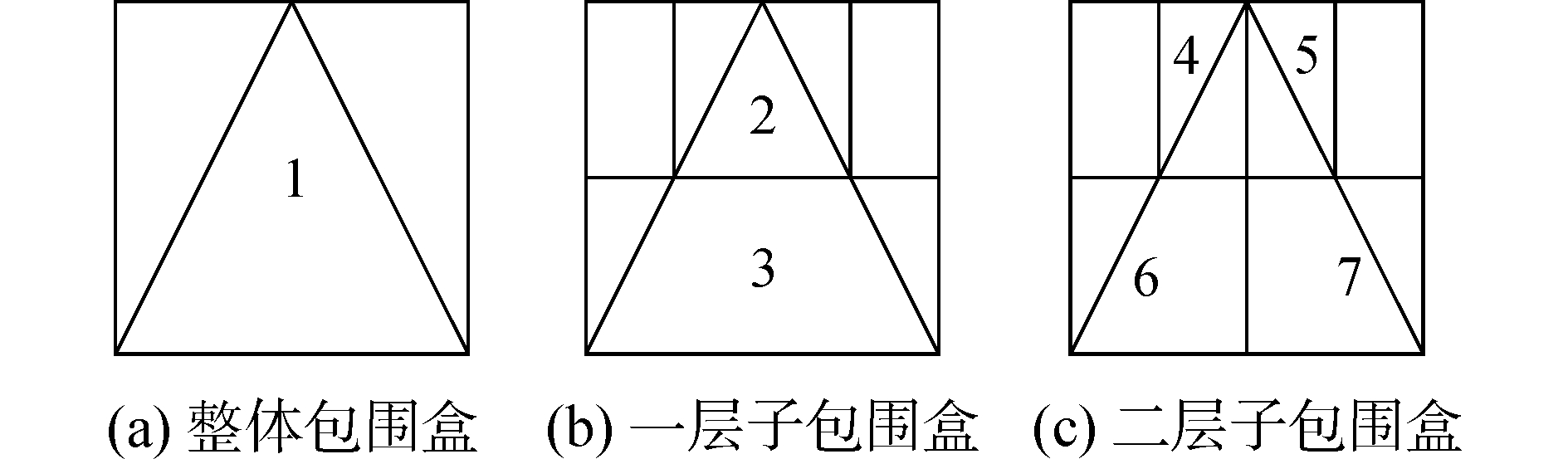Download: 图 4 包围盒构建图 Fig. 4 The structure of hierarchical bounding box

2.2 质点转换

 ${x_j} = \frac{{{x_i}}}{s}$ (2)
 ${y_j} = \frac{{{y_i}}}{s}$ (3)
 ${z_j} = \frac{{{z_i}}}{s}$ (4)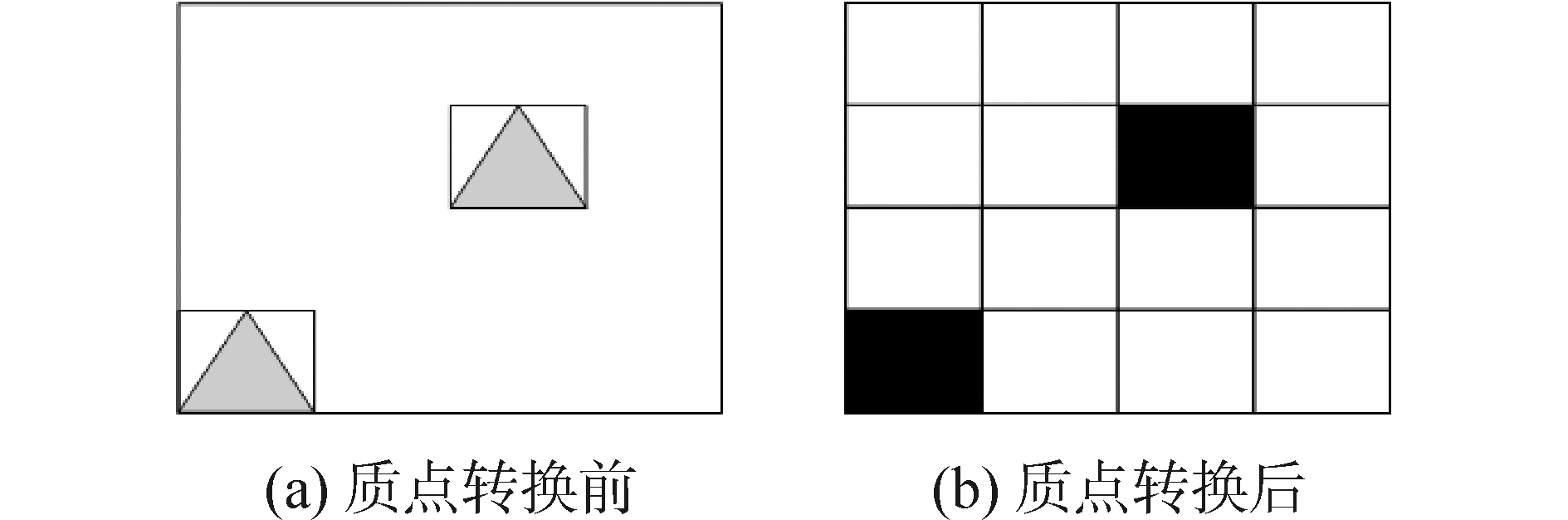Download: 图 5 质点转换图 Fig. 5 Particle conversion

2.3 质点还原

 ${L_{xi}} = \frac{{|{x_{{i_1}}} - {x_{i{a_1}}}{\rm{|}}}}{s} + \frac{{{\rm{|}}{x_{{i_2}}} - {x_{i{a_2}}}|}}{s}$ (5)
 ${L_{yi}} = \frac{{|{y_{{i_1}}} - {y_{i{a_1}}}{\rm{|}}}}{s} + \frac{{{\rm{|}}{y_{{i_2}}} - {y_{i{a_2}}}|}}{s}$ (6)
 ${L_{zi}} = \frac{{|{z_{{i_1}}} - {z_{i{a_1}}}{\rm{|}}}}{s} + \frac{{{\rm{|}}{z_{{i_2}}} - {z_{i{a_2}}}|}}{s}$ (7)

 ${L_{xj}} = |{x_{{j_1}}} - {x_{{j_2}}}|$ (8)
 ${L_{yj}} = |{y_{{j_1}}} - {y_{{j_2}}}|$ (9)
 ${L_{zj}} = |{z_{{j_1}}} - {z_{{j_2}}}|$ (10)

1) 选择空间中相同大小包围盒数目相对较多的物体将其包围盒定义为单位包围盒；

2) 根据选择好的单位包围盒，确定质点转换中所需的转换系数；

3) 根据确定好的转换系数，利用质点转换公式把空间中包围盒全部转换为空间中的一个点；

4) 对空间中运行的点进行还原距离的计算；

5) 对空间两点的间0距进行计算；

6) 最后通过对两点间还原距离和间距之间的比较判断该质点是否还原为包围盒。

2.4 OBB层次包围盒相交测试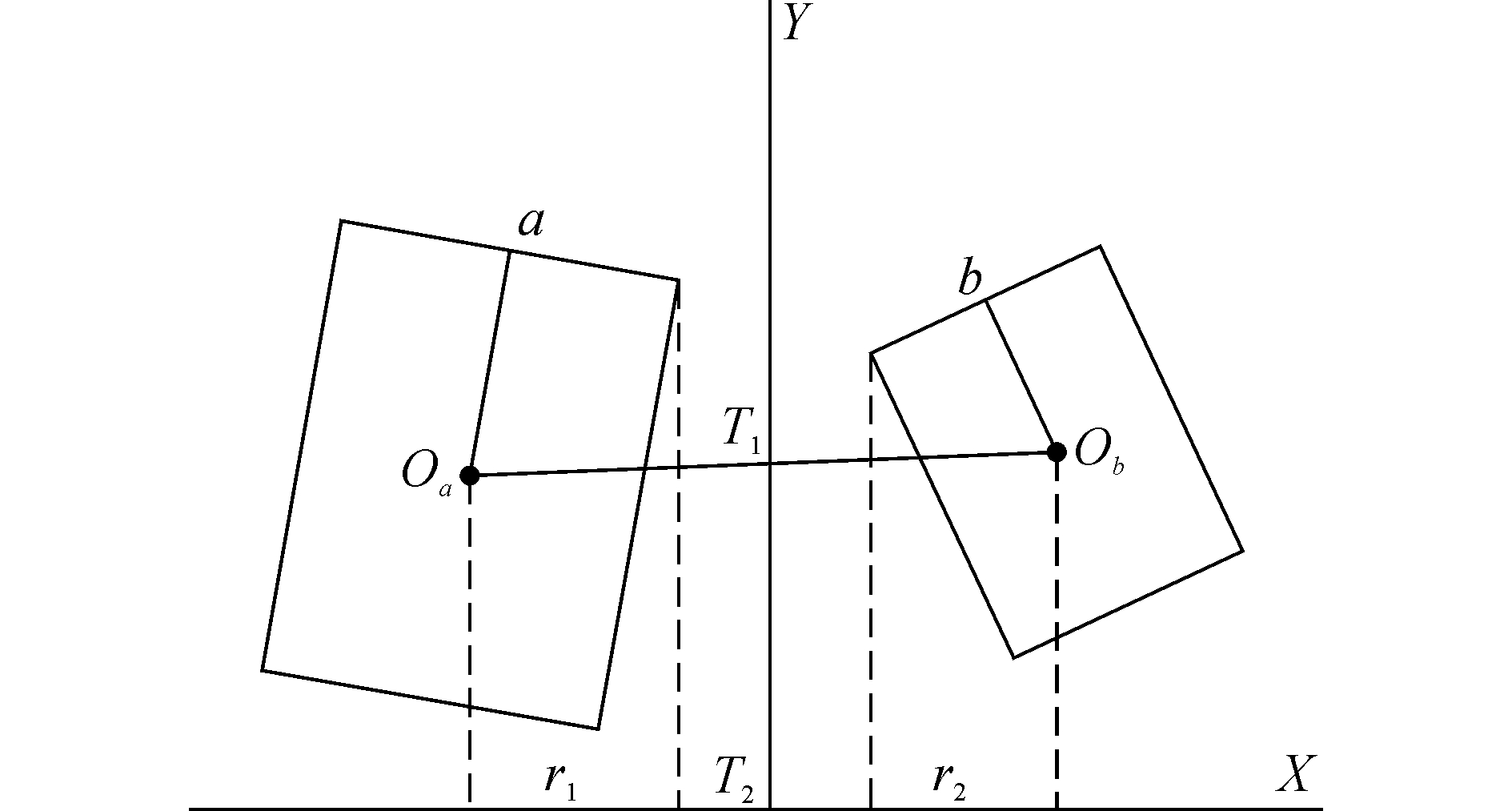Download: 图 6 分离轴定理图 Fig. 6 Separation axis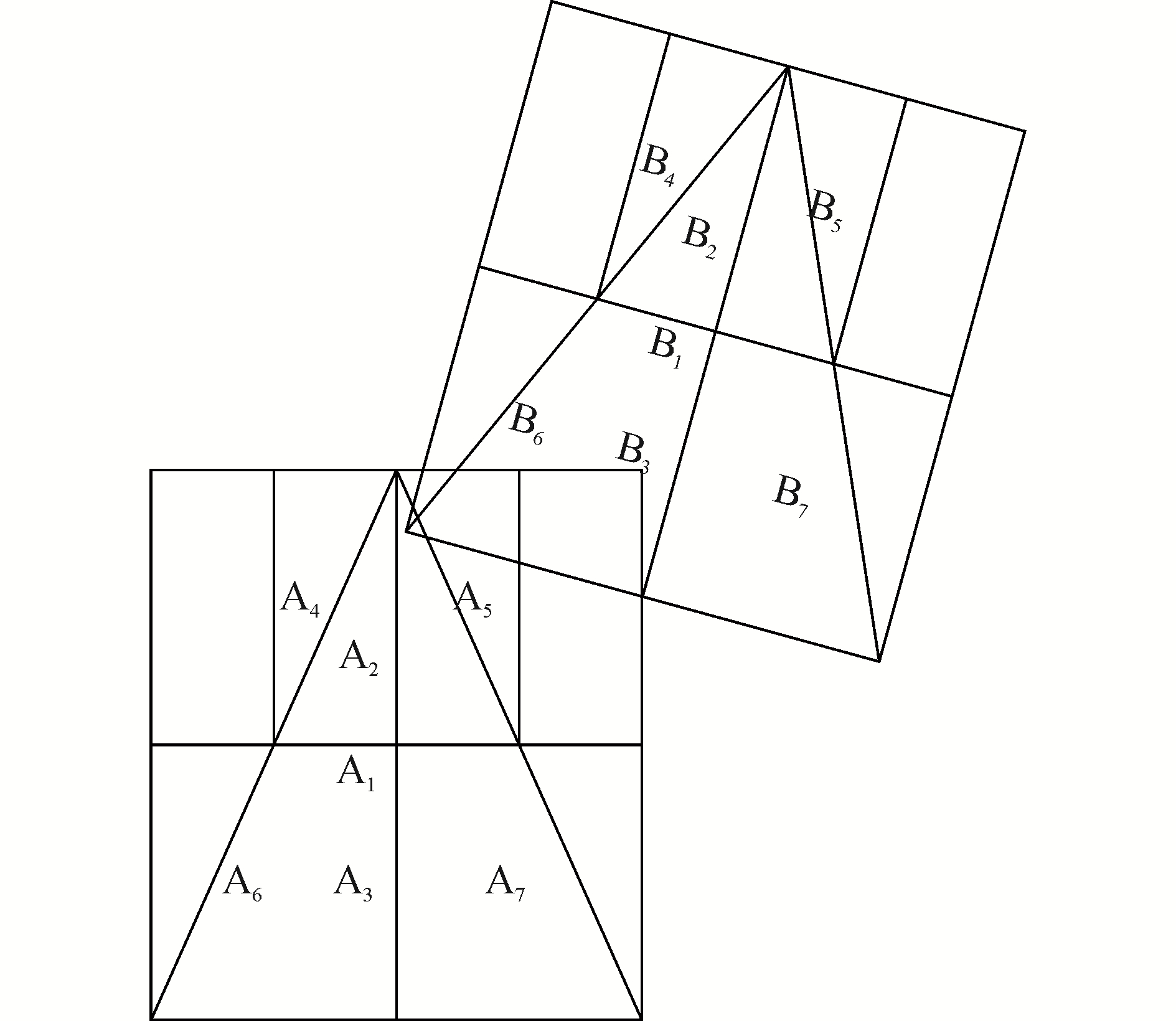Download: 图 7 层次包围盒检测图 Fig. 7 Detection of hierarchical bounding boxes

1) 对物体A的最外层包围盒A1和物体B的最外层包围盒B1进行分离轴定理相交测试，测试结果两包围盒相交。

2) 使A1和物体B的第一层子包围盒B2、B3进行相交测试，测试结果A1和B3相交。

3) 使A1和物体B的第一层子包围盒中的B3的子包围盒B6、B7进行相交测试，测试结果A1和B6相交，因为图中为两层子包围盒的层次包围盒所以经过三次测试确定本次碰撞的物体B的终端节点包围盒B6

4) 根据确定的终端节点包围盒B6对物体A的层次包围盒进行遍历，首先使B6和物体A的第一层子包围盒A2、A3进行相交测试，测试结果B6和A2相交。

5) 使B6和物体A的第一层子包围盒中的A2的子包围盒A4，A5进行相交测试，测试结果B6与A5相交，那么可以确定本次碰撞的物体A的最终节点包围盒A5

6) 通过前面相互遍历的相交测试能够知道两物体的最终节点包围盒B6和A5相交，那么就可以判定两物体发生了碰撞。

3 实验结果与分析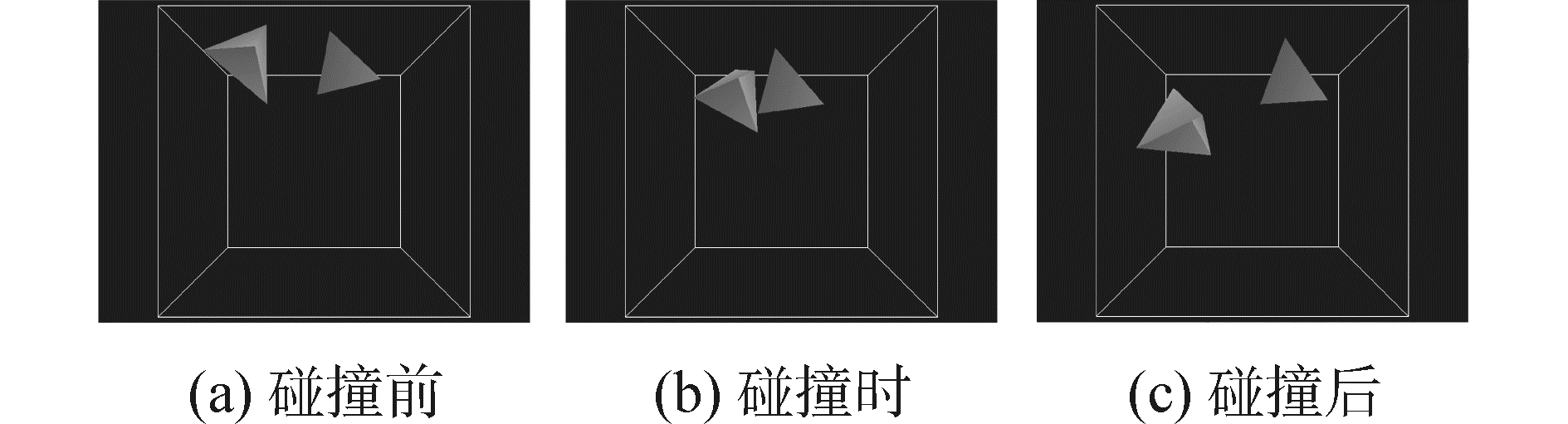Download: 图 8 两物体碰撞图 Fig. 8 Two objects collide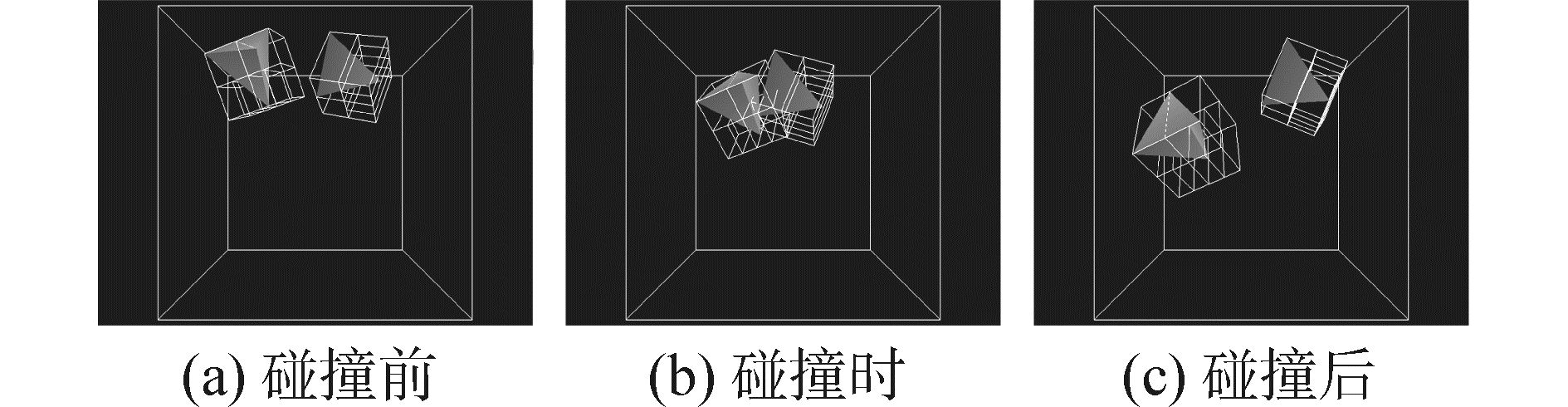Download: 图 9 显示包围盒的两物体碰撞图 Fig. 9 Two objects collide with bounding boxes表 1 两物体花费时间 Tab.1 The time consumption of two objects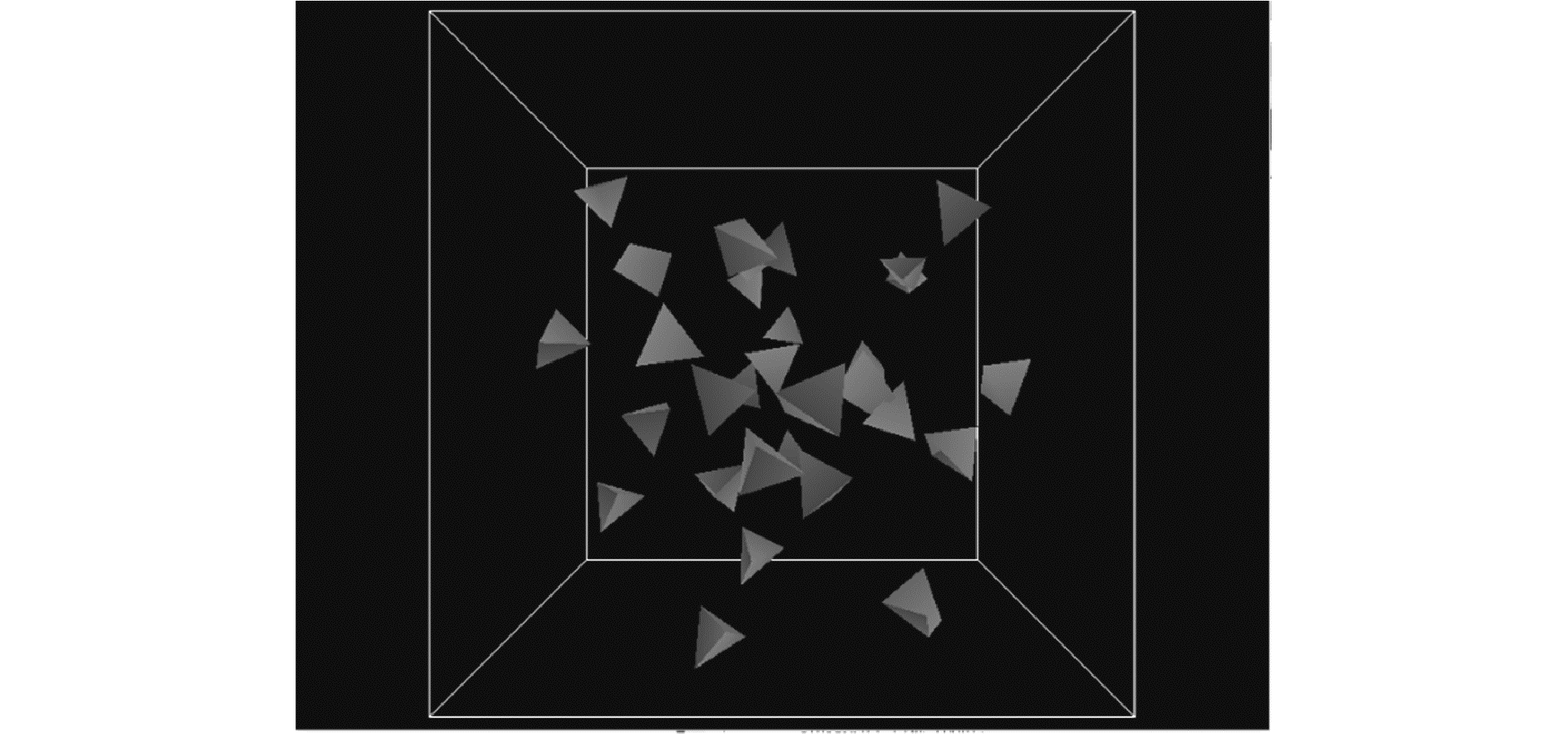Download: 图 10 多物体碰撞图 Fig. 10 Multiple objects collide表 2 区域中心包围盒法花费时间 Tab.2 The time consumption of region center bounding box method表 3 质点转换和包围盒混合算法花费时间 Tab.3 The time consumption of particle conversion and bounding box hybrid algorithm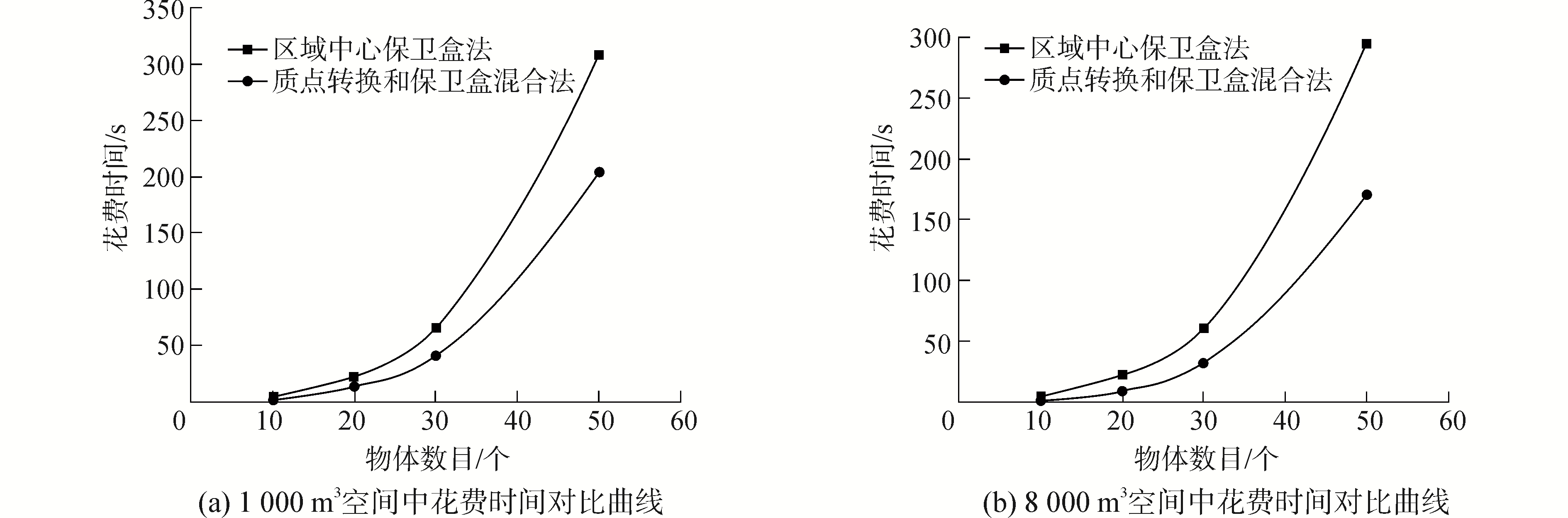Download: 图 11 花费时间对比曲线 Fig. 11 Curves of consumption time
4 结论

1) 通过在传统OBB包围盒算法基础上利用区域中心法构建包围盒，然后在该包围盒的基础上与质点转换的方法相结合来进行碰撞检测，通过实验结果显示该方法能够保障检测的精度。

2) 在多物体环境中对两种算法进行了效率对比实验，通过实验结果可以看出，比起前人算法质点转换和包围盒相结合的方法能够有效地减少碰撞检测所花费的时间，检测效率更高，随着空间大小的增加检测效率还会进一步提高，特别适用于空间中存在大量物体的复杂环境。

  于凌涛, 王涛, 宋华建, 等. 面向虚拟手术的碰撞检测优化算法[J]. 哈尔滨工程大学学报, 2014, 35(9): 1164-1170. YU Lingtao, WANG Tao, SONG Huajian, et al. An optimization algorithm of collision detection applied to virtual surgery[J]. Journal of Harbin Engineering University, 2014, 35(9): 1164-1170. (0)  WONG S K, LIN W C, HUANG C H, et al. Radial view based culling for continuous self-collision detection of skeletal models[J]. ACM transactions on graphics, 2013, 32(4): Article No. 114. (0)  SCHVARTZMAN S C, PÉREZ Á G, OTADUY M A. Star-contours for efficient hierarchical self-collision detection[J]. ACM transactions on graphics, 2010, 29(4): 80. (0)  TANG Min, MANOCHA D, YOON S E, et al. VolCCD:fast continuous collision culling between deforming volume meshes[J]. ACM transactions on graphics, 2011, 30(5): 111. (0)  TANG Min, MANOCHA D, KIM Y J. Hierarchical and controlled advancement for continuous collision detection of rigid and articulated models[J]. IEEE transactions on visualization and computer graphics, 2014, 20(5): 755-766. DOI:10.1109/TVCG.2013.266 (0)  OUYANG Fan, ZHANG Tie. Octree-based spherical hierarchical model for collision detection[C]//Proceedings of the 10th World Congress on Intelligent Control and Automation. Beijing, China, 2012: 3870-3875. http://ieeexplore.ieee.org/document/6359118/ (0)  HE Liang, ORTIZ R, ENQUOBAHRIE A, et al. Interactive continuous collision detection for topology changing models using dynamic clustering[C]//Proceedings of The 19th Symposium on Interactive 3D Graphics and Games. San Francisco, USA, 2015: 47-54. http://www.ncbi.nlm.nih.gov/pubmed/26191116 (0)  PABST S, KOCH A, STAßER W. Fast and scalable CPU/GPU collision detection for rigid and deformable surfaces[J]. Computer graphics forum, 2010, 29(5): 1605-1612. DOI:10.1111/cgf.2010.29.issue-5 (0)  WANG Huamin. Defending continuous collision detection against errors[J]. ACM transactions on graphics, 2014, 33(4): 122. (0)  BROCHU T, EDWARDS E, BRIDSON R. Efficient geometrically exact continuous collision detection[J]. ACM transactions on graphics, 2012, 31(4): 96. (0)  TANG Min, TONG Ruofeng, WANG Zhendong, et al. Fast and exact continuous collision detection with Bernstein sign classification[J]. ACM transactions on graphics, 2014, 33(6): 186. (0)  张智, 邹盛涛, 李佳桐, 等. 凸多面体碰撞检测的棱线投影分离算法[J]. 计算机辅助设计与图形学学报, 2015, 27(8): 1407-1415. ZHANG Zhi, ZOU Shengtao, LI Jiatong, et al. A collision detection algorithm between convex polyhedrons based on projection of edges[J]. Journal of computer-aided design & computer graphics, 2015, 27(8): 1407-1415. DOI:10.3969/j.issn.1003-9775.2015.08.007 (0)  林凌, 张明敏, 潘志庚, 等. 布料人体快速连续碰撞检测与响应[J]. 软件学报, 2015, 26(S2): 1-7. LIN Ling, ZHANG Mingmin, PAN Zhigeng, et al. Image based detection and response of continuous fast collision between cloth and human body[J]. Journal of software, 2015, 26(S2): 1-7. (0)  XING Dengpeng, XU De, LIU Fangfang. Collision detection for blocking cylindrical objects[C]//Proceedings of 2015 IEEE/RSJ International Conference on Intelligent Robots and Systems. Hamburg, Germany, 2015: 4798-4803. http://ieeexplore.ieee.org/xpl/articleDetails.jsp?arnumber=7354051 (0)  史旭升, 乔立红, 朱作为. 基于改进OBB包围盒的碰撞检测算法[J]. 湖南大学学报(自然科学版), 2014, 41(5): 26-31. SHI Xusheng, QIAO Lihong, ZHU Zuowei. Algorithm of collision detection based on improved oriented bounding box[J]. Journal of Hunan University (natural sciences), 2014, 41(5): 26-31. (0)